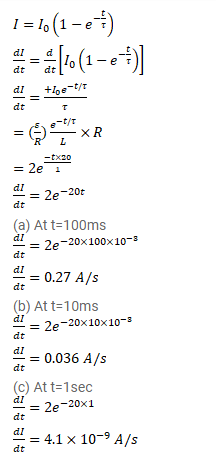# An LR circuit has L=0.1H and R=20Ω.

Question:

An $L R$ circuit has $L=0.1 H$ and $R=20 \Omega$. It is connected across an emf of $2.0 \mathrm{~V}$ at $t=0$. Find di/dt at

(a) $t=100 \mathrm{~ms}$,

(b) $t=200 \mathrm{~ms}$ and

(c) $t=1.0 \mathrm{~s}$.

Solution: